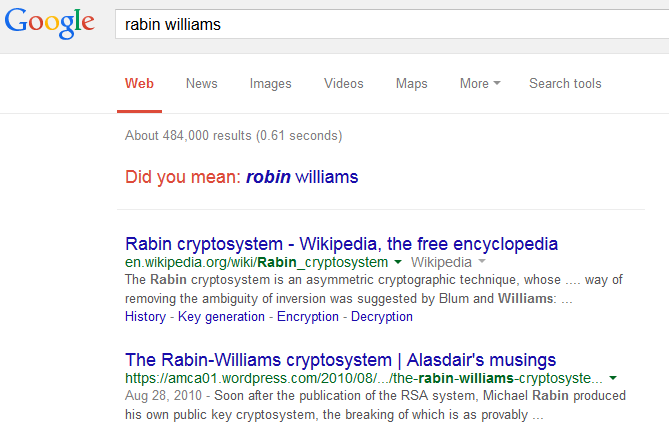# Random Rabin-Williams signatures

Last weekend I was sent a paper on a vulnerability in an implementation of Rabin-Williams signatures. Or, as Google suggests, Robin Williams signatures.Rabin-Williams is an asymmetric cryptosystem of which the security depends on the fact that given a number N which is the product of two large primes p and q (so N = p·q), it is very hard to actually find p and q. This is also what lies behind the security of RSA; in fact Michael Rabin is the ‘R’ from RSA (edit: he is not, of course, he just happens to share the first letter of his surname with Ron Rivest).

Moreover, Rabin-Williams also uses the fact that it is easy to take a square root modulo N, assuming one knows p and q (and especially if certain conditions about p and q are met), but without this knowledge it is impossible to do in practice (as long as p and q are sufficiently large). Squaring a number modulo N — which is of course the inverse of taking a square root — is trivial, even without knowledge of p and q.

So in Rabin-Williams, signing or decrypting a message involves taking a square root (which is thus only possible if one knows the private key), while verifying a signature or encrypting a message involves squaring a number, which anyone can do as the public key is, indeed, public. Rabin-Williams is especially useful in cases where the speed of the signing or encrypting operation is important.

There is a caveat though: taking a square root modulo N doesn’t give a unique answer. That is less surprising than one might think: taking the square root of 9 in the integers, that is finding a number whose square is 9, gives both 3 and –3 as solutions.

In the case we’re in here, there are in fact four square roots that occur in two pairs: s, –s and t, –t. When decrypting a message, one needs some extra information about the message to know which of the four square roots is the correct one. In practice, one might know enough about the message to determine the ‘correct’ square root, while there are also some mathematical ‘fixes’ to ensure the correct square root is always returned.

That signatures aren’t unique isn’t a problem in itself. After all, a signature is merely a proof of something (possession of the private key together with the message) and all that matters it that the receiver can cryptographically verify that the sender did indeed sign the message.

This is all fine if the signing algorithm always returns the same signature — and of course, algorithms have a nice tendency to do that. But there is a big hole one might fall into: knowing one square root in each of the pairs (so s or –s and t or –t) makes it trivial to compute p and q and thus to crack the cryptosystem. (It happens that the difference of these roots, viewed as integers, shares a non-trivial divisor with N.)

So it is essential that an adversary never gets hold of multiple signatures (square roots). And this is what goes wrong in the Crypto++ library: a fix to prevent timing attacks introduces a randomness in the computation of the square roots, which means that the algorithm outputs each possible square root with a probability of 1/4.

If an adversary is thus able to have the same message signed twice, they are able to crack the private key with a probability of 1/2. (This probability approaches 1 as more signatures are generated.) This is a serious weakness.

It was discovered by Yandex researcher Evgeny Sidorov and was published by IACR this week. If you like cryptography and number theory, I strongly recommend you read the paper. Evgeny also provides a simple fix to the implementation.

While admitting that I am far from an expert in this field, I have become a bit wary of Rabin-Williams and the fact that there are four square roots. Mathematics is strong enough to fix all the potential issues. But as always, people remain a big liability. And in the end, it is people who will have to implement the algorithms.